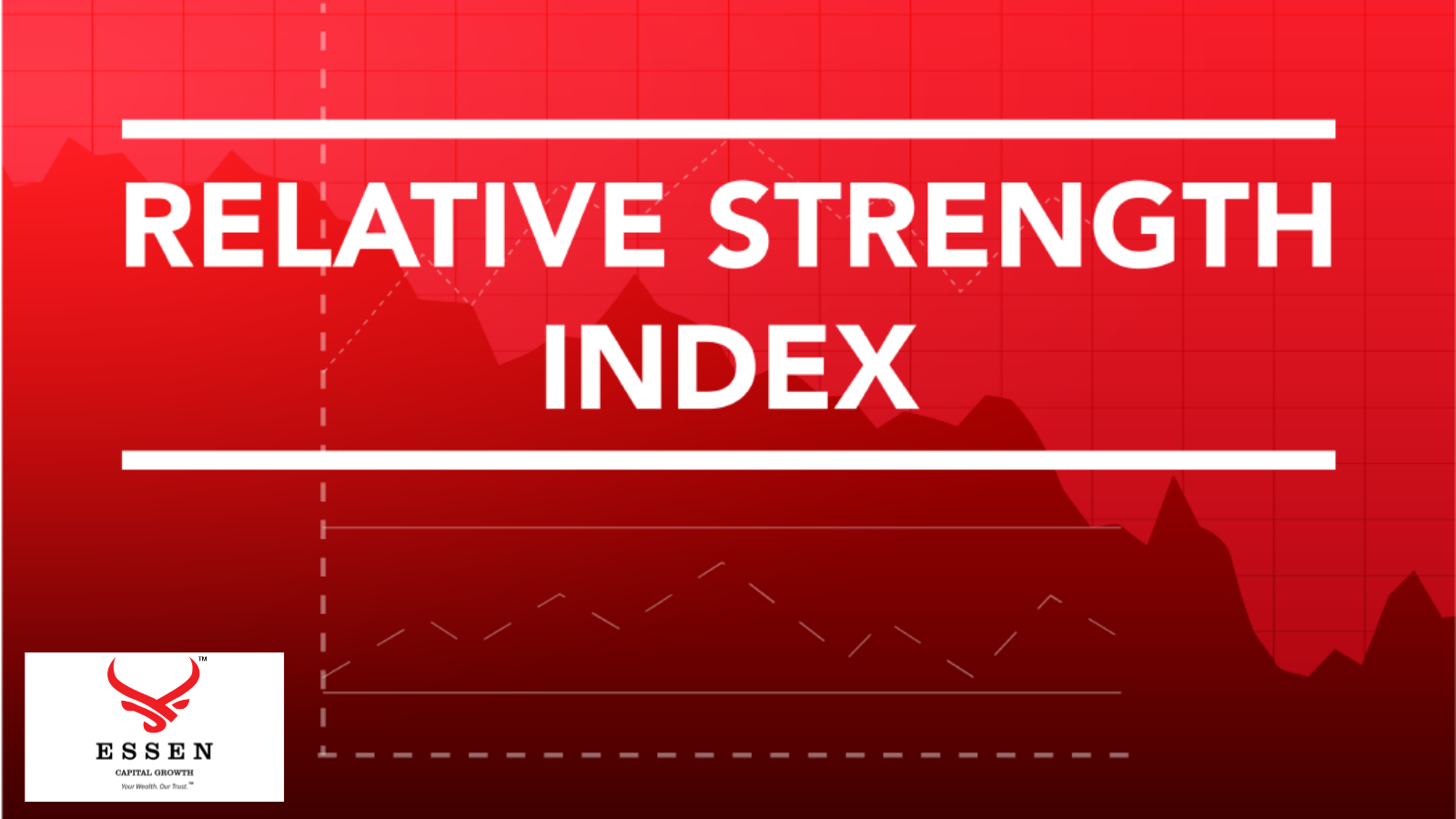# Relative Strength Index

June 02, 2021

Relative Strength Index

DEFINITION:

Relative Strength Index (acronym RSI) is one of the most extensively used momentum oscillators in the realm of technical analysis of stocks. It was introduced by Welles Wilder in June 1978 and its computation is explained in detail in his book New Concepts in Technical Trading System. Momentum oscillator measures the velocity and magnitude of price movements of a security. RSI compares the magnitude of average gains and average losses of a security for drawing inferences about its strength and weakness over a predetermined time period.DESCRIPTION:

Relative Strength is computed using the formula

RSI = 100 – (100/1+RS)

RS (Relative Strength) = Average of X days up closes / Average of X days down closes

Wilder used the 14-day RSI, which is still the most commonly used RSI. However, an analyst is free to decide the number of days for computing the same.

For example, during a 14-day trading period assume that a security has generated positive returns on 9 days and negative returns on 5 days. In this case, the Relative Strength Index is calculated as follows

1. Calculate the absolute gain in each of the 9 up days. Add the absolute gain of each day and divide by 14. This will give the figure of average up closes.

2. Calculate the absolute loss in each of the 5 days. Add the absolute loss of each day and divide by 14. This will give the figure of average down closes.

3. Dividing the average up closes or gains by the average down closes or losses gives us the Relative Strength.

4. The figure is further normalized using the above formula so as to ensure that it lies between 0 and 100.

For computing the next RSI, the following steps are followed

1. For calculating the next average gains, multiply the previous average gains by 13 and add today’s gains if any and divide the result by 14. In our previous example, we will multiply the average absolute gains of 9 days and add today’s absolute gain (if any) and divide the result by 14.

2. For calculating the next average losses, multiply the previous average losses by 13 and add today’s loss if any and divide the result by 14. In our example, we will multiply the average absolute losses of 5 days and add today’s absolute loss (if any) and divide by 14.

3. The next step involves dividing the average gains by the average losses for getting the figure of Relative Strength.

4. Finally, the RSI is found using the formula RSI = 100 – (100/1+RS)

The following inferences can be drawn from Relative Strength Index

1. Overbought and Oversold levels: RSI gives an indication of the impending reversals or reaction in price of a security. RSI moves in the range of 0 and 100. So an RSI of 0 means that the stock price has fallen in all of the 14 trading days. Similarly, an RSI of 100 means that the stock price has risen in all of the 14 trading days. In technical analysis, an RSI of above 70 is considered an overbought area while an RSI of less than 30 is considered as an oversold area. RSI can be used as a leading indicator as it normally tops and bottoms ahead of the market, thereby indicating an imminent correction in the price of a security. It is pertinent to note that the levels of 70 and 30 needs to be adjusted according to the inherent volatility of the security in question.

2. Failure Swings: This is also called support and resistance penetrations or breakouts. Failure swings are indications of impending market reversals.

Bearish                                                     Failure                                                 SwingIn this chart, the RSI touches the 70 marks, the threshold of overbought zone then falls to 60. This is called the failure point. Post that, it moves again but rises less than the previous high of 70, thereby, creating a failure swing. It then falls below the failure point there creating a bearish failure swing. It acts as a signal for the trader to go for a short position in the security.

Bearish                                                 Failure                                                      SwingIn this chart, the RSI touches the 30 marks, the threshold of oversold zone and then rises to 40, the failure point. It then falls again but the fall is lower than the previous value of 30. Moreover, the RSI crosses the failure point to rise even higher thereby creating a bullish failure swing. It acts as a signal for a trader to go for a long position in the security.

1.     Divergence:

Divergence results when the price of a security and the RSI behave differently. If the security price is decreasing or flat but RSI is increasing, it’s a sign of divergence. Similarly, when the security price is increasing or flat by RSI is falling, it’s a sign of divergence. It is a strong indication of an imminent market correction.

2.     Support and Resistance:

In some situations, the RSI exhibits support and resistance levels with more clarity than the security price itself.

The 14-day RSI of stock prices of Reliance Power in NSE. The overbought level set at over 70 and oversold level set at below 30.According to Wilder, the RSI used together with bar chart can provide pivotal inputs to an analyst for drawing inferences from a chart.

Source: https://economictimes.indiatimes.com/definition/relative-strength-index